# Solar Panel Size Calculator and Charts

Optional: If left blank, we’ll use a default value of 50% DoD for lead acid batteries and 100% DoD for lithium batteries.
Estimated solar panel size:
0.00 watts

## How to Calculate the Size of Solar Panel I Need

To determine how many solar panels you need with our solar calculator, enter the following in their given fields:

• Battery depth of discharge
• Battery capacity in Ah
• Battery voltage
• Battery type
• Charge time (peak sun hours)
• Solar charge controller type

Then click on calculate.

### Example 1

Say you have a solar energy system with a 12v 50Ah lithium-ion battery bank, an MPPT charge controller, and a depth of discharge of 100%. If you want your solar system to charge the batteries fully within 7 peak sun hours, your system size would be approximately 110 watts.

Example 2

If you have a 12v 80Ah lead-acid battery bank with a depth of discharge of 50%. The solar system size needed to get a full charge within 8 peak sun hours when using a PWM charge controller would be approximately 130 watts.

## Solar Panel Size Chart for a 12v Battery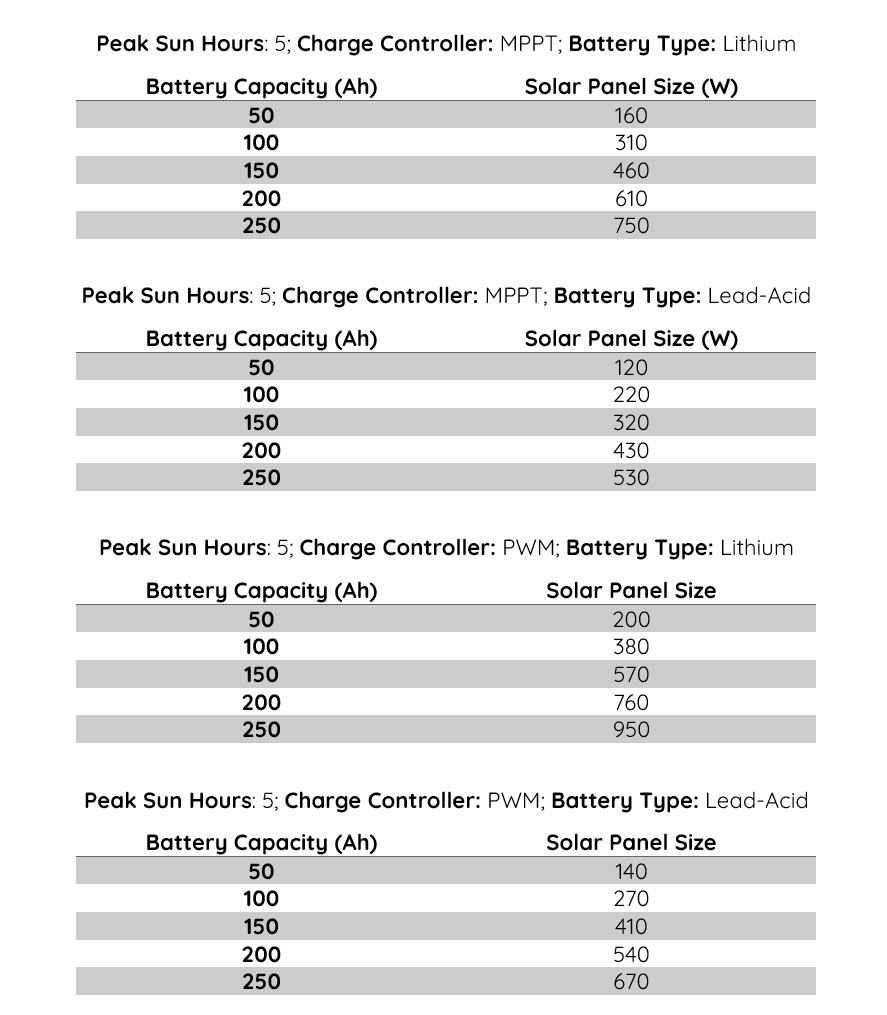## Solar Panel Size Chart for a 24v Battery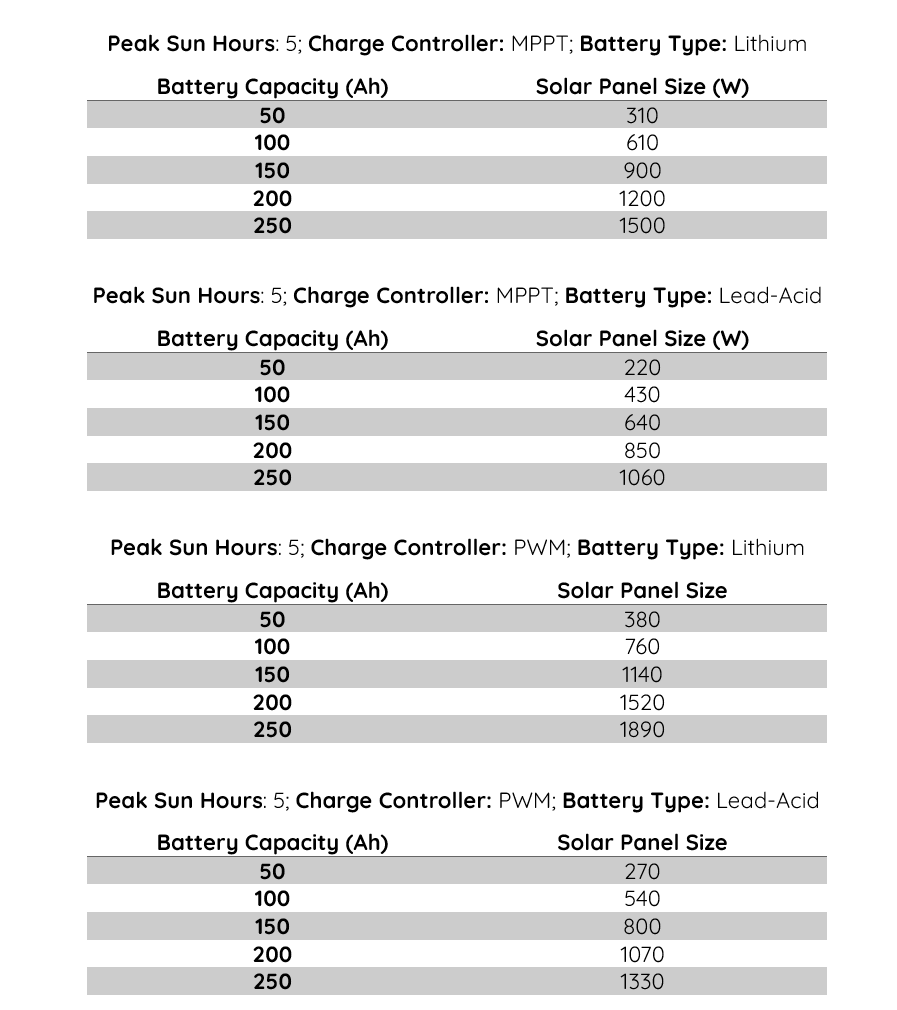## Solar Panel Size Chart for 100 Ah Battery Bank## Solar Panel Size Chart for 120 Ah Battery Bank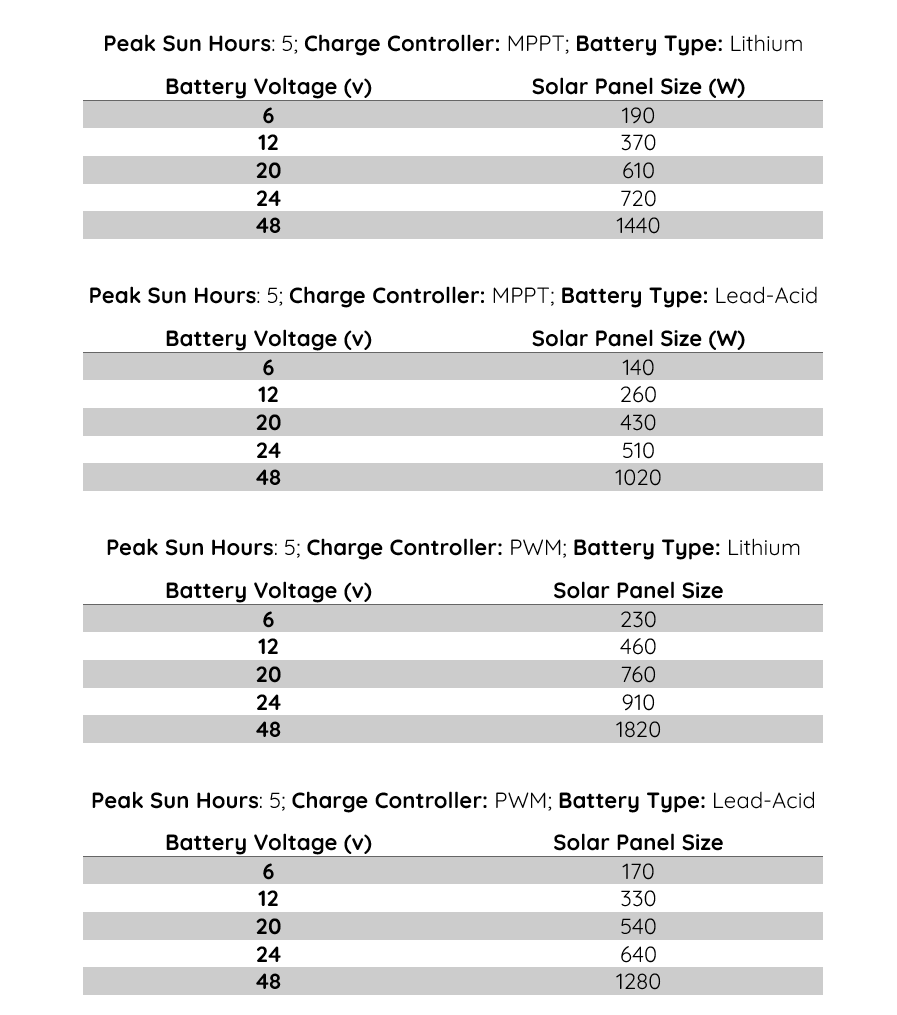## Solar Panel Size Chart for 150 Ah Battery Bank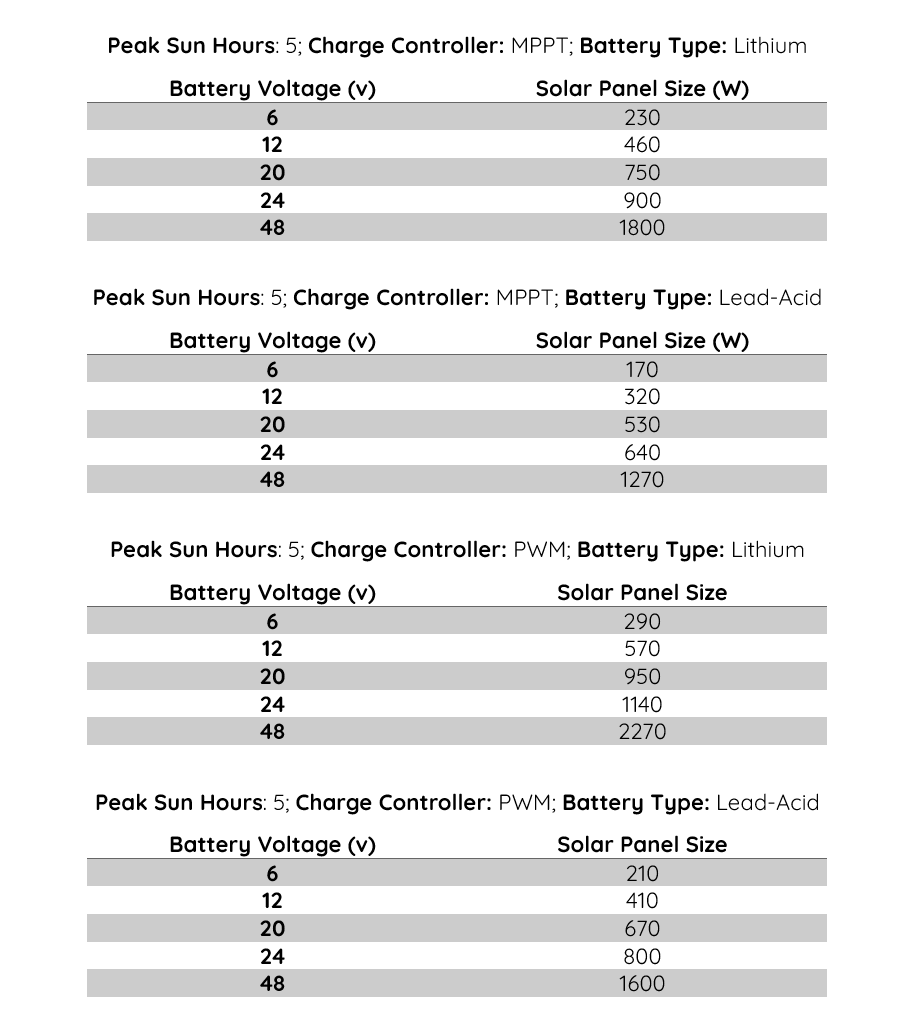## Solar Panel Size Chart for 200 Ah Battery Bank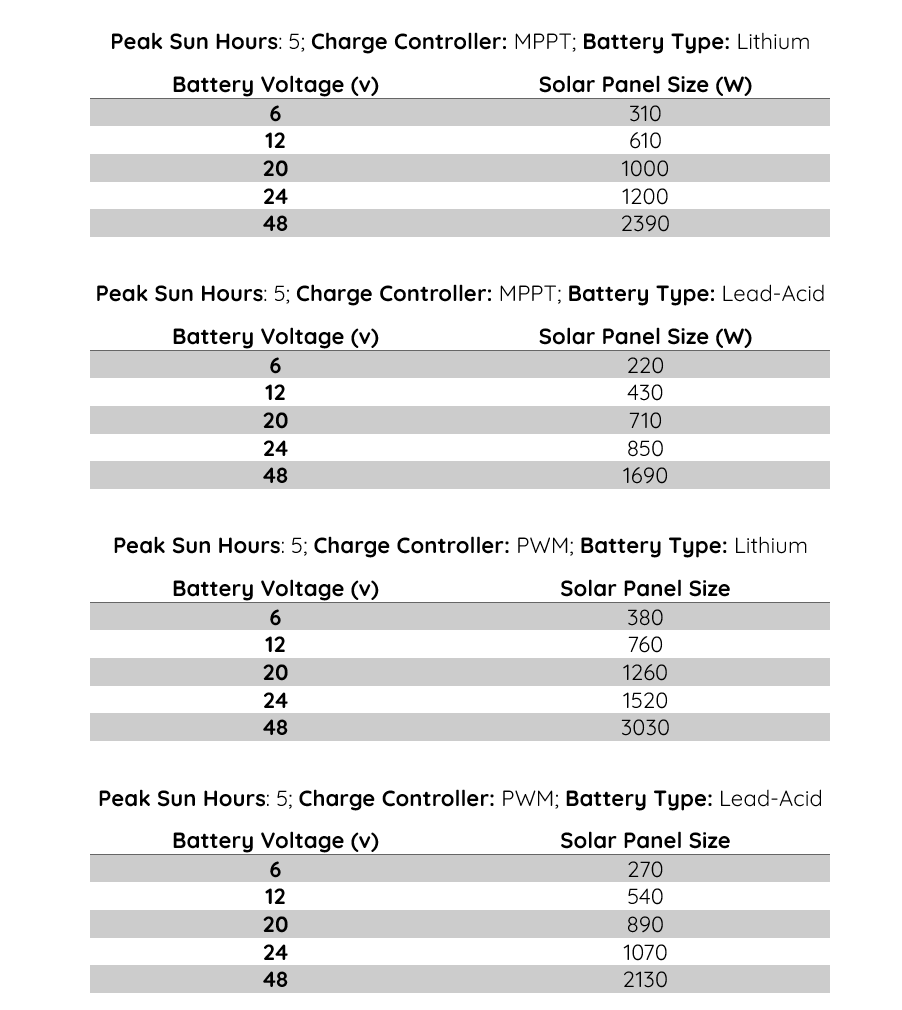The average peak sun hours in the United States is 5. So, in all charts, we used peak sun hours of 5.

## Factors Affecting the Size of Solar Panel Array Needed for Your System

When trying to calculate the size of the solar panels you need for your solar system, you must consider the following factors:

### Battery’s Depth of Discharge

A battery’s depth of discharge (DOD) is a percentage indicator of how much of the total battery capacity a battery has discharged at a given time. Going by this, when a battery’s DOD is 0%, the battery is not discharged at all (fully charged). Then when the DOD is at 100%, the battery is fully discharged.

Why is your battery’s depth of discharge important when calculating solar array size? Well, with a higher level of discharge, your solar panel system will need more power to give your battery a full charge.

### Battery Voltage

The battery voltage plays a part in determining how much solar power the battery needs to charge fully within peak sun hours.

For one, the solar panel voltage must at least match the battery voltage. So, since the voltage is directly proportional to power, it checks out that batteries with higher voltages need more solar power to charge within shorter peak sun hours.

Basically, the higher your battery voltage, the higher the solar array size needed.

### Battery Capacity (Ah)

The battery capacity in Ah signifies how much charge the battery needs to get a full charge. As expected, with a higher battery capacity, you need a larger solar power system to get a full charge within shorter peak sun hours.

Basically, your battery capacity affects solar array size and your preferred charging time in peak sun hours.

### Battery Type

Different types of batteries have different charging patterns under different circumstances. So, when doing an estimate of the ideal solar panel array size for your battery bank, the solar panel calculator considers the charging pattern of the battery.

### Solar Charge Controller Type

Solar charge controller type is another factor that determines solar array size.

Based on the charging technique, there are two main types of charge controllers: Pulse Width Modulation (PWM) and Maximum Power Point Tracking (MPPT).

Of both options, MPPT offers higher charging efficiency than PWM charge controllers. So, an MPPT charge controller typically needs less power to fully charge your battery bank. On the flip side, a PWM may need slightly more power to charge the battery bank.

Going by the above, the number of solar panel arrays needed for a PWM system may be slightly more than that for an MPPT system if other factors are the same.

If you are going for a large solar panel system, an MPPT solar charge controller is the better option. But for a small system, you may opt for a PWM charge controller.

When choosing the charge controller for a small system, you may have to consider solar panel’s cost. If the solar panels and other fixtures are not too expensive, you may opt for an MPPT over a PWM.

### Charge Time (in Peak Sun Hours)

The effect of charge time on solar system size is pretty straightforward. For faster charge times, you’ll need many solar panels. But if regular charge times are okay for you, you may not necessarily go for a very large solar panel size.

Basically, if your area has long peak sun hours, you can work with a moderate system size. But if your region gets a short duration of peak sun hours, you need a significantly large system size to ensure your battery bank gets a full charge within time.

## What Are Peak Sun Hours?

Peak sun hours is the number of hours solar intensity averages 1000 watts per square meter. In other words, one peak sun hour equals 1 hour of sunlight at 1000 W/m² of solar irradiance. In other words, one peak sun hour is 1000 Wh/m² of insolation.

### Why Is Peak Sun Hour Important?

The intensity of the sun reaching solar panels determines how much power they generate. With higher solar intensity (solar irradiance), solar panels generate more power. But with lower intensity, they generate less.

If we were to calculate solar panel size using sunlight duration, our estimate might be off. Since the intensity of the sun typically varies greatly throughout the day, solar panel power generation estimated using the duration of exposure to sunlight will vary greatly. But with a standard like peak sun hours, our estimates are closer to the actual thing.

## How to Figure Out Your Average Peak Sun Hours Per Day

As we’ve already demonstrated, peak sun hours are essential when trying to determine the ideal solar array size for your solar system. However, it varies from one region to another. So, how can you figure out the average peak sun hours per day in your region?

### Using a Solar Resource Map

If you live in the United States, this map from the National Renewable Energy Laboratory shows the annual global horizontal solar irradiance of every state.

The National Renewable Energy Laboratory also offers other solar irradiance data, including monthly average solar irradiance.

### Calculating Peak Sun Hours Using Solar Irradiance Data

You can also calculate peak sun hours yourself if you have irradiance data for your region. For instance, if the data below demonstrates the irradiance in your area between 12 pm and 5 pm:

• 900 W/m² – 12 pm
• 600 W/m² – 1 pm
• 700 W/m² – 2 pm
• 100 W/m² – 3 pm
• 400 W/m² – 4 pm
• 200 W/m² – 5 pm

The total irradiance between the given period would be 2900 W/m². In peak sun hours, it would be 2900/1000 = 2.9 peak sun hours. So, in your area, you’ll get around 2.9 peak sun hours between 12 pm and 5 pm.

When trying to figure out your average peak sun hours per day, work with global horizontal irradiance or global tilted irradiance.

### Determine Solar Panel Size Using Peak Sun Hours and Energy Usage

In situations where you do not have a battery yet or do not know the ratings of your battery, you can calculate how many panels you need with the values of your average energy usage, solar panel efficiency, and peak sun hours.

For instance, say your average energy usage is 300 kWh per month, and the average peak sun hour in your region is 5 hours per day. If you are getting solar panels with 25% efficiency, your solar panel size would be as follows:

First, we’ll calculate your daily energy usage from the monthly energy usage:

Daily energy usage = 300 kWh/30 = 10 kWh per day

Next, we’ll divide the daily energy usage by the average peak sun hours to get estimated watts of the solar array:

Estimated wattage of the array = 10/5 = 2 kW

Now, we’ll adjust the estimated wattage of the array using the efficiency of the panels:

Adjusted wattage of the array = 2 + (2 x 25%) = 2 + (2 x 0.25) = 2 + 0.5 = 2.5 kW

We can also rewrite the adjustment as:

Adjusted wattage of the array = 2 x 125% = 2 x 1.25 = 2.5 kW

The estimated total watts of the PV array you’ll need is 2.5 kW (2500 W). But to determine how many solar panels you need, you’ll have to divide those total watts by the watts of one panel. So, if each solar panel is 100 W, you’ll need 2500/100 = 25 solar panels.

### How to Estimate How Much Energy Your Electronics Consume on Average

You might be able to determine your average monthly kWh usage from your electric bills. Then once you have that, you can divide by 30 to get your daily energy usage.

If you are only moving a fraction of your electricity bill to the solar system, you’ll have to multiply your daily usage by that fraction. For instance, if you only want 30% of your load on the solar system, you’ll multiply your daily usage by 0.3.

If you do not intend to use the same load from your electric bills on the solar energy system, you’d have to calculate the energy usage for the intended solar load.

Calculating the energy usage of your electronics is pretty straightforward; you only need two values – power and duration of use in hours.

For instance, if the data below shows the devices in your solar load, their wattages, and daily duration of use, here’s what your daily energy usage would be:

• 15 W Light Bulb on for 10 hours per day
• 10 W Router on 24 hours per day
• 1000 W Coffee machine on for 1 hour per day
• 50 W LED TV on for 6 hours per day
• 300 W Freezer on for 16 hours per day
• 65 W Laptop – 4 hours per day

Daily energy usage = (15 x 10) + (10 x 24) + (1000 x 1) + (50 x 6) + (300 x 16) + (65 x 4)

= 150 + 240 + 1000 + 300 + 4800 + 260

= 7010 Wh per day

= 7010/1000 = 7.01 kWh per day

If the watts (power rating) of some devices are not available, you can multiply their voltage and current to get their watts.